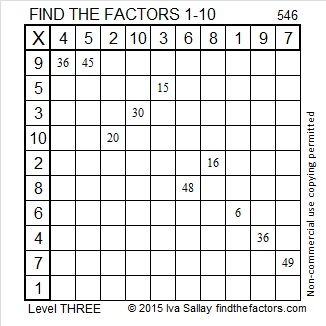# 546 and Level 3

Contents

### A Math Vocabulary Puzzle:

Puzzles can be a great resource in teaching mathematics. I’ve made crossword puzzles to review vocabulary words before, but none of them looked as inviting to complete as the one Resourceaholic recommended in her 7 July 2015 post on end of term resources. What a difference a little clip art makes to a well-constructed crossword puzzle! The post also includes links to several other amazing mathematics-related puzzles including a polygon word search and a number sequence number search puzzle. Check it out!

### Today’s Puzzle:Print the puzzles or type the solution on this excel file: 10 Factors 2015-07-06

A Logical Approach to solve a FIND THE FACTORS puzzle: Find the column or row with two clues and find their common factor. Write the corresponding factors in the factor column (1st column) and factor row (top row).  Because this is a level three puzzle, you have now written a factor at the top of the factor column. Continue to work from the top of the factor column to the bottom, finding factors and filling in the factor column and the factor row one cell at a time as you go.### Factors of 546:

• 546 is a composite number.
• Prime factorization: 546 = 2 x 3 x 7 x 13
• The exponents in the prime factorization are 1, 1, 1, and 1. Adding one to each and multiplying we get (1 + 1)(1 + 1)(1 + 1)(1 + 1) = 2 x 2 x 2 x 2 = 16. Therefore 546 has exactly 16 factors.
• Factors of 546: 1, 2, 3, 6, 7, 13, 14, 21, 26, 39, 42, 78, 91, 182, 273, 546
• Factor pairs: 546 = 1 x 546, 2 x 273, 3 x 182, 6 x 91, 7 x 78, 13 x 42, 14 x 39, or 21 x 26
• 546 has no square factors that allow its square root to be simplified. √546 ≈ 23.36664### Sum-Difference Puzzle:

546 has eight factor pairs. One of the factor pairs adds up to 85, and a different one subtracts to 85. If you can identify those factor pairs, then you can solve this puzzle!### More about the Number 546:

546 is made from 3 consecutive numbers so it is divisible by 3.

The sum of all the prime numbers from 53 to 83 is 546. Can you list all those prime numbers?

546 is the hypotenuse of the Pythagorean triple 210-504-546. Those three numbers have eight common factors, but what is the greatest common factor?

This site uses Akismet to reduce spam. Learn how your comment data is processed.